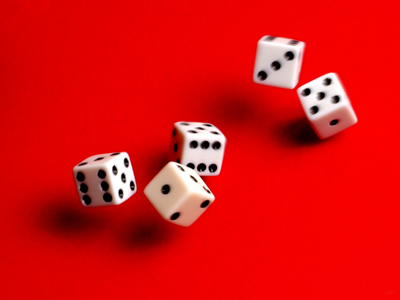The number 6 is all around us. For example, dice have 6 sides.

# 6 Times Table

In KS1 Maths and Numeracy children will be exposed to the times tables as early as possible in Year 1. The 1, 2, 5 and 10 times tables are taught first before the 3, 4, 6, 7, 8 and 9 times tables follow. By the end of Year 2 children are often fluent in their times tables up to 10.

This quiz will test children's familiarity with their six times table, which will help them in studying maths in Years 1 and 2.

Do you know your six times table? See how many you can get right.

1.
10 x 6 =
66
54
16
60
Remember, to multiply a number by 10 just add a 0 to the end of it
2.
7 x 6 =
40
48
42
36
If you know your 5 times table you could work out 5 x 7 = 35 and then add 7 to get 6 x 7 = 42
3.
6 x 2 =
8
12
18
24
6 x 2 is the same as 6 + 6
4.
0 x 6 =
12
1
6
0
5.
6 x 1 =
6
1
7
0
6.
6 x 8 =
40
56
48
44
7.
5 x 6 =
24
30
36
25
8.
9 x 6 =
60
56
54
52
9.
3 x 6 =
24
12
9
18
3 x 6 is the same as 6 + 6 + 6
10.
6 x 6 =
36
30
42
12
You could work out 5 x 6 = 30 and then add 6 to find 6 x 6 = 36
Author:  Graeme Haw